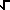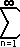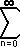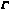Home    |    Teacher    |    Parents    |    Glossary    |    About UsEmail this page to a friendResources· Cool Tools · Formulas & Tables · References · Test Preparation · Study Tips · Wonders of MathSearchRoot Expansions (Math | Calculus | Series | Root)

Expansions of the Root Functions
Function Expansion Summation(x)

(0<=x<=2)

=  (1/0!) + [1/2] (1/1!) (x-1) + [(1/2) (-1/2)] (1/2!) (x-1)2 + [(1/2) (-1/2) (-3/2)] (1/3!) (x-1)3 + [(1/2) (-1/2) (-3/2) (-5/2)] (1/4!) (x-1)4 + ...

= 1 + (1/2)(x-1) - (1/8)(x-1)2 + (1/16)(x-1)3 - (5/128)(x-1)4 + ...

Very awkwardly expressed:
 1+(-1)n-1 n (2n-2)! (x-1)n 22n-1 (n!)2
or:(3/2) (x-1)n(3/2 - n) n!

1 /(x)

(0<x<=2)

=  (1/0!) + [-1/2] (1/1!) (x-1)1 + [(-1/2)(-3/2)] (1/2!) (x-1)2 + [(-1/2)(-3/2)(-5/2) (1/3!) (x-1)3 + ...

= 1 - (1/2)(x-1) + (3/8)(x-1)2 - (5/16)(x-1)3 + (35/128)(x-1)4 -  ...

Very awkwardly expressed:(-1)n (2n)! (x-1)n  22n (n!)2
or:(1/2) (x-1)n(1/2 - n) n!